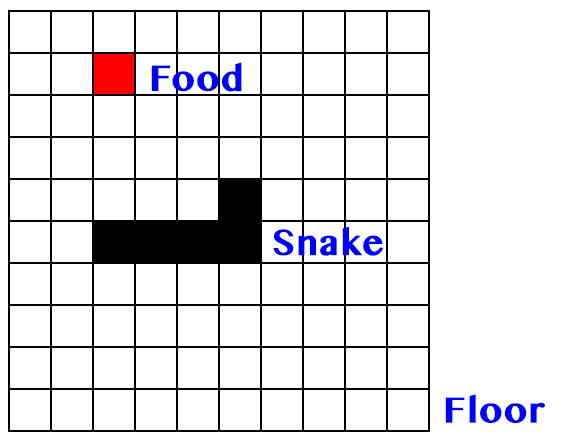## TypeScript & CoffeeScript

1. TypeScript 是 JavaScript 的超集，这使得它能够与普通的 JavaScript 混用，而 CoffeeScript 使用自己的那一套类 Ruby 的语法使得这是不可能滴;
2. TypeScript 玩的概念比较多，比如 Module, Interface 等，而 CoffeeScript 自己实现的语法糖则比较多；
3. TypeScript 已经开始支持 ES6，CoffeeScript 会不会支持和什么时候支持，还都是未知数；
4. 最大的一点不同：TypeScript 在编译过程中可对类型进行检查，将 JavaScript 这个灵活的动态型语言变成了静态类型的语言。算是有利有弊吧：好处是相当于将部分的“测试”工作提前了，问题的定位也更加精准；坏处是丧失了一点灵活性与增加了一些代码量。

## TypeScript & Go

TypeScript 在某些方面与 Go 很像，是我喜欢的部分（也许是我接触的静态型语言实在太少）。比如定义一个取两数较大值的函数：

Go:

``````func max(a, b int) int {
if a > b {
return a
}
return b
}
``````

TypeScript:

``````function max(a: number, b: number): number {
if (a > b) {
return a
}
return b
}
``````

Go 的自定义类型：

``````type Human struct {
name string
age int
phone string
}

var person Human
``````

TypeScript 的 Type Interface：

``````interface Human {
name: string
age: number
phone: string
}

var person: Human
``````

## 贪食蛇1. 地板: Floor
2. 蛇: Snake
3. 食物: Food

1. Floor: 白色
2. Snake: 黑色
3. Food: 红色

``````const FLOOR = {
SPACE: 'space',
BODY: 'body',
FOOD: 'food'
}
``````
``````.space {
background-color: white;
}

.body {
background-color: black;
}

.food {
background-color: red;
}
``````

``````interface Block {
pos: Pos
type: string
node: HTMLElement
}
``````

``````interface Pos {
x: number
y: number
}
``````

``````class Floor {
private table: HTMLTableElement
private parent: HTMLElement
private row: number
private col: number
public blocks: Block[] // 提供给 Snake 使用的 block 集合

constructor(options?) {
options = options || {};
this.table = document.createElement('table')
this.parent = options.parent || document.body
this.row = options.row || 20
this.col = options.col || 20
this.blocks = []
}

initialize() {
let x: number
let y: number

for (y = 0; y < this.row; y++) {
let tr = <HTMLTableRowElement>this.table.insertRow(-1)
for (x = 0; x < this.col; x++) {
let td = <HTMLTableCellElement>tr.insertCell(-1)
td.className = FLOOR.SPACE
this.blocks.push({
node: td,
type: FLOOR.SPACE,
pos: {x: x, y: y}
})
}
}

this.parent.appendChild(this.table)
}
}
``````

``````class Snake {
private initLength: number
private bodies: Block[]
private speed: number

constructor(options?) {
options = options || {}
this.initLength = options.initLength || 3
this.speed = options.speed || 300
this.bodies = []
}

born() {
for (let i = this.initLength - 1; i >= 0; i--) {
this.bodies.push(floor.blocks[i]) // floor 是 Floor 的一个实例
}

this.bodies.forEach(body => {
body.type = FLOOR.BODY
body.node.className = body.type // 着色
})
}
}
``````

``````let snake = new Snake()
snake.born()
``````

``````class Snake {
...
move() {
let head: Block = this.bodies
let tail: Block = this.bodies[this.bodies.length - 1]
let next: Block = this.sbling(head) // 获取 head 右侧的 block

// body move
for (let i = this.bodies.length - 1; i > 0; i--) {
this.bodies[i] = this.bodies[i - 1]
}

next.type = FLOOR.BODY
this.bodies = next

// clear original tail
tail.type = FLOOR.SPACE
tail.node.className = tail.type

// change color of blocks
this.blocks.forEach(block => {
block.node.className = block.type
})
}

sbling(source: Block): Block {
return this.blocks.filter((block) => {
if (source.pos.x + 1 === block.pos.x
&& source.pos.y === block.pos.y) {
return true
}
})
}
}
``````

``````born() {
...
// keep moving
setInterval(function() { this.move(); }.bind(this), this.speed)
}
``````

``````const enum Direction {
left, up, right, down
}

class Snake {
...
private direction: Direction
private offsets: Array<number[]>

constructor(options?) {
...
this.direction = Direction.right
this.offsets = [[-1, 0], [0, -1], [+1, 0], [0, +1]]
}
...

move() {
let head: Block = this.bodies
let tail: Block = this.bodies[this.bodies.length - 1]
let next: Block = this.sbling(head, this.direction)
...
}

sbling(source: Block, direction: Direction): Block {
return this.blocks.filter((block) => {
if (source.pos.x + this.offsets[direction] === block.pos.x
&& source.pos.y + this.offsets[direction] === block.pos.y) {
return true
}
})
}
}
``````

``````born() {
...
let keyHandler = (e: KeyboardEvent): void  => {
const keyCode: number = e.keyCode || e.which || e.charCode

switch (keyCode) {
case KeyCode.left:
if (this.direction !== Direction.right) {
this.direction = Direction.left
}
break
case KeyCode.up:
if (this.direction !== Direction.down) {
this.direction = Direction.up
}
break
case KeyCode.right:
if (this.direction !== Direction.left) {
this.direction = Direction.right
}
break
case KeyCode.down:
if (this.direction !== Direction.up) {
this.direction = Direction.down
}
break
}
}

document.addEventListener('keydown', keyHandler, false)
}
``````

``````class Floor() {
...
genFood() {
// 在地板内的随机位置
let pos: Pos = {
x: Math.floor(Math.random() * this.col),
y: Math.floor(Math.random() * this.row)
}

// 根据位置获取食物 block
let food = this.blocks.filter((block) => {
if (block.pos.x === pos.x && block.pos.y === pos.y) {
return true
}
})

food.type = FLOOR.FOOD
food.node.className = food.type
}
}
``````

``````class Snake {
...
born() {
...

// generate food
floor.genFood()

// keep moving
setInterval(function() { this.move(); }.bind(this), this.speed)
}

move() {
...

if (next.type === FLOOR.FOOD) {
this.eat(next)
}

// body move
for (let i = this.bodies.length - 1; i > 0; i--) {
this.bodies[i] = this.bodies[i - 1]
}

...
}

eat(block: Block) {
this.bodies.push(block)
floor.genFood()
}
}
``````

``````move() {
let head: Block = this.bodies
let tail: Block = this.bodies[this.bodies.length - 1]
let next: Block = this.sbling(head, this.direction)

if (!next || next.type === FLOOR.BODY) {
this.die()
return
}

if (next.type === FLOOR.FOOD) {
this.eat(next)
}

...
}
``````

## 总结

``````this.blocks[[x, y]] = {
node: td,
type: FLOOR.SPACE,
pos: [x, y]
}
``````

``````this.blocks
this.blocks[this.blocks.length - 1]

this.blocks[[1, 2]]
this.blocks[this.blocks.pos]
``````